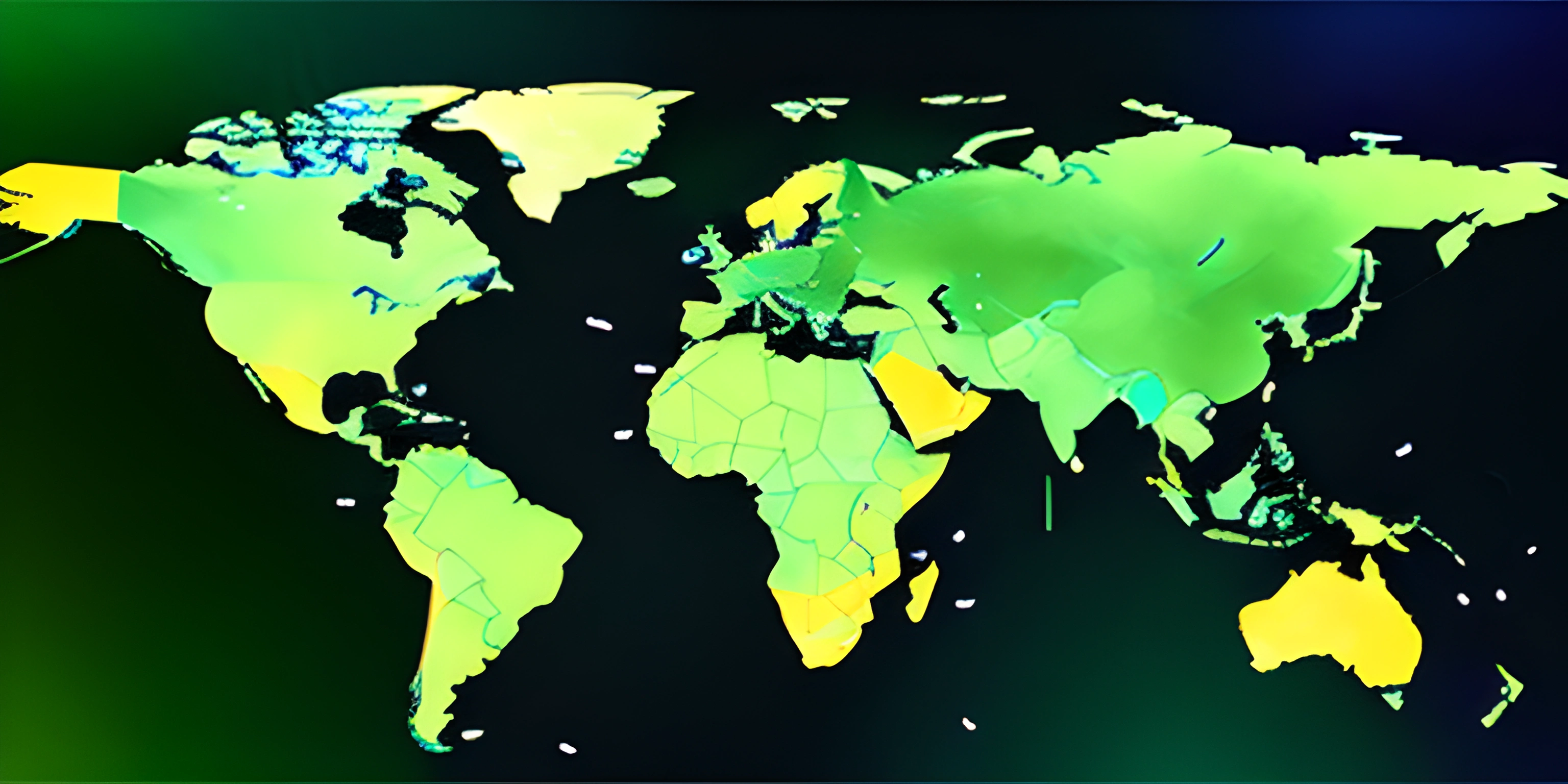# Techniques for Solving the Traveling Salesperson ProblemNote: this page has been created with the use of AI. Please take caution, and note that the content of this page does not necessarily reflect the opinion of Cratecode.

The Traveling Salesperson Problem (TSP) is a classic conundrum that has tickled the brains of computer scientists and mathematicians alike for centuries. The challenge: given a list of cities and the distances between each pair of cities, find the shortest possible route that visits every city exactly once and returns to the origin city. Doesn't sound too tough, right? Well, buckle up, because the TSP is a notorious example of an NP-hard problem, meaning it can be quite the computational doozy.

Fear not! Even though the TSP is a challenging problem, there are several techniques to tackle it, ranging from exact solutions to approximation algorithms. Let's dive into some of these strategies and see how they fare in finding the shortest path for our enthusiastic salesperson.

## Brute Force

When in doubt, brute force it out! A brute-force algorithm generates all possible permutations of the cities, calculates the total distance for each permutation, and then selects the shortest path. This approach guarantees we'll find the optimal solution, but it comes with a hefty price tag: its time complexity is O(n!), where n is the number of cities. As the number of cities increases, the computational time required explodes, rendering this method impractical for large instances of TSP.

## Backtracking

Backtracking is a refined version of brute force that seeks to reduce the search space by eliminating partial solutions that clearly won't lead to an optimal result. The algorithm proceeds depth-first through the decision tree (formed by the cities) and backtracks whenever a partial solution is deemed infeasible. While the worst-case time complexity still hovers around O(n!), backtracking is generally more efficient than brute force, especially for small to medium-sized instances of TSP.

## Dynamic Programming

Enter the world of dynamic programming, where we take advantage of overlapping subproblems to optimize our solution. The Held-Karp algorithm is a dynamic programming method that solves the TSP in O(n^2 * 2^n) time, a significant improvement over brute force and backtracking for larger instances. The algorithm's trade-off is its O(n * 2^n) space complexity, meaning it requires a substantial amount of memory.

## Approximation Algorithms

When exact solutions become too computationally expensive, we can turn to approximation algorithms. These techniques provide near-optimal solutions with a fraction of the computation time. One such method is the Christofides algorithm, which guarantees a solution within 3/2 times the optimal route. By combining minimum spanning trees, perfect matchings, and Eulerian circuits, the Christofides algorithm provides a relatively efficient and effective approach to tackling larger instances of TSP.

In conclusion, the TSP is a fascinating problem with a variety of solution techniques, each with its own strengths and weaknesses. Depending on the problem size and specific requirements, one may choose between brute force, backtracking, dynamic programming, or approximation algorithms to help our salesperson find the most efficient path. Happy traveling!

## FAQ

### What is the Traveling Salesperson Problem?

The Traveling Salesperson Problem (TSP) is a classic optimization challenge in computer science. It involves a salesperson who needs to visit a set of cities, with the goal of finding the shortest possible route that visits each city exactly once and returns to the starting city. TSP is known as an NP-hard problem, meaning it is computationally complex, and finding an optimal solution becomes increasingly difficult as the number of cities increases.

### What are some common techniques to solve the Traveling Salesperson Problem?

There are various strategies and techniques for solving the TSP, including:

1. Brute Force: This involves checking all possible routes and selecting the shortest one. However, this method becomes impractical as the number of cities increases, due to the computational complexity.
2. Greedy Algorithm: This technique involves selecting the nearest unvisited city for each step, which might not always result in the optimal solution.
3. Dynamic Programming: Using a bottom-up approach, it breaks the problem into smaller overlapping subproblems, and stores the results to avoid redundant calculations.
4. Genetic Algorithm: Inspired by natural selection, this method uses a population of candidate solutions and evolves them through selection, crossover, and mutation to find an optimal solution.
5. Simulated Annealing: A probabilistic optimization algorithm that simulates the annealing process in metallurgy to find an optimal solution.

### Can the Traveling Salesperson Problem be solved optimally in polynomial time?

Currently, there is no known algorithm that can solve TSP optimally in polynomial time. TSP is an NP-hard problem, which means that it is computationally complex and finding an optimal solution becomes increasingly difficult as the number of cities increases. However, research continues to discover new algorithms and techniques that can potentially solve TSP faster or with better approximations.

### How does the Greedy Algorithm work for solving the Traveling Salesperson Problem?

The Greedy Algorithm is a simple technique for solving the TSP that follows a "greedy" approach. The algorithm starts at a particular city, and for each step, it selects the nearest unvisited city as the next destination. Once all cities are visited, the algorithm returns to the starting city. While it can provide a quick solution, the Greedy Algorithm might not always result in the optimal route, as it makes local decisions without considering the global implications.

### What are some real-world applications of the Traveling Salesperson Problem?

Although the TSP is often framed as a salesperson visiting cities, its applications extend far beyond that. Real-world applications of the TSP include:

• Vehicle routing for delivery services or garbage collection
• Scheduling and routing for airline or transportation networks
• Circuit board drilling optimization in electronics manufacturing
• DNA sequencing and protein folding in bioinformatics
• Tour planning for sightseeing or field service technicians Understanding and solving the TSP can lead to significant efficiency improvements and cost savings in various industries.